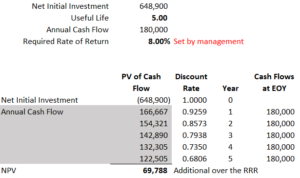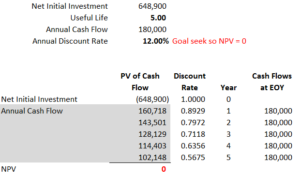Calculating Net Present Value and the Internal Rate of Return In Excel

# Calculating Net Present Value and the Internal Rate of Return In Excel

Net Present Value and Internal Rate of Return are important terms for the non-financial manager to understand. I want to do a series on basic tools used by the finance professional to explain what they use often, how they’re used, and why they’re important to people outside of the trade. This is a useful exercise for anyone because it helps enforce their understanding of them as they progress into upper management where these terms are used often in evaluating capital asset acquisitions. For this post I wanted to explore the use of Net Present Value (NPV) and Internal Rate of Return (IRR) when evaluating fixed asset purchases.

To the non-finance or accounting person this may sound like the least interesting concept on earth. However, the extraordinary value lies in establishing an “apples to apples” comparison when allocating large pools of money. Investments in fixed assets are necessary to extend the capability of any organization whether it be through expansion or an attempt to increase productivity, but as we all know, resources are finite and we have to ensure we’re getting the best bang for our bucks.

The first concept I want to share is calculating the Net Present Value of a potential source of cash flow. NPV is used to compare incoming cash flows versus a Required Rate of Return (RRR). The RRR is a benchmark set by management that can either reflect the cost of funds to borrow in a leveraged organization or the expected rate of return if the firm were to instead invest these monies into a pool of assets, such as stock or bonds or some combination therein. This helps paint a picture of the investment’s performance relative to interest paid or returns realized passively from the market. Below is an example of this calculation that might help you understand the concept better.

For our potential fixed asset purchase, we’re expecting an outflow of 648,900 but a return over 5 years of 180,000. Management has told us that our Required Rate of Return for an investment is 8.00%. To calculate the flows relative to this amount we will need to multiply each year by a discount rate. You may recall your finance class days when you had to research the appendix for a table to provide this rate, however, we can just use Excel to do it. In this example we’ll take 1/((1.08)^(# of years)) and multiply each line item by that figure by creating a formula.

This gives us a NPV of 69,788 positive cash flow over the period. Not a terrible return over the RRR, but not a terrific one either.A second tool we use is one where we back into the Annual Discount Rate to determine the Internal Rate of Return (IRR) on the investment. For our example, our 648,900 investment will provide us a 12.00% return over the life of the asset. We calculate this using the forumulas we established in the example above, but instead use Goal Seek in Excel to derive the Annual Discount Rate by forcing the NPV = 0. In this example the IRR is 12.00%, which is a modest return on investment.I hope you found this explanation helpful. This is something you’ll come across in your career if you find yourself in a finance or accounting role or on a board position where you’re weighing out proposals against your investment pool.